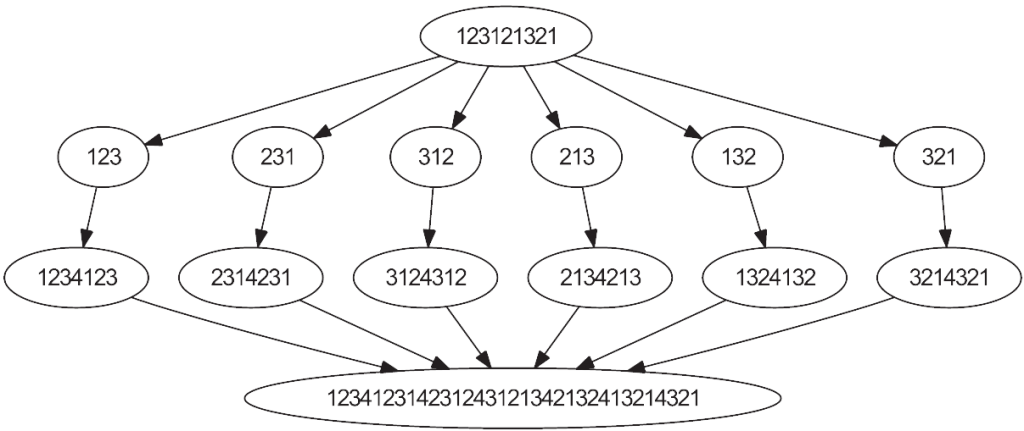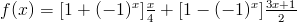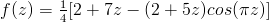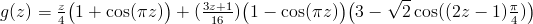### Archive

Posts Tagged ‘Open problems’

## “Obvious” Does Not Imply “True”: The Minimal Superpermutation Conjecture is False

August 22nd, 2014

One of my favourite examples of an “obvious” mathematical statement that is actually false is the “fact” that if$R,S,T \subseteq \mathbb{R}^n$ are vector spaces then$\dim(R + S + T) = \dim(R) + \dim(S) + \dim(T) \\ {}_{{}_{{}_{.}}}\quad\quad\quad\quad\quad\quad\quad\quad - \dim(R\cap S) -\dim(R\cap T) -\dim(S\cap T) \\ {}_{{}_{{}_{.}}}\quad\quad\quad\quad\quad\quad\quad\quad+\dim(R\cap S\cap T).$

The reason that the above statement seems so obvious is that the similar fact$\dim(R + S) = \dim(R) + \dim(S) - \dim(R\cap S)$ does hold, so it’s very tempting to think  “inclusion-exclusion, yadda yadda, it’s simple enough to prove that it’s not worth writing down or working through the details”. However, it’s not true: a counterexample is provided by 3 distinct lines through the origin in$\mathbb{R}^2$.

There is another problem that I’ve been thinking about for quite some time that is also “obvious”: the minimal superpermutation conjecture. This conjecture was so obvious, in fact, that it appeared as a question in a national programming contest in 1998. Well, last night Robin Houston posted a note on the arXiv showing that, despite being obvious, the conjecture is false .

### Superpermutations

What is the shortest string that contains each permutation of “123” as a contiguous substring? It is straightforward to check that “123121321” contains each of “123”, “132”, “213”, “231”, “312”, and “321” as substrings (i.e., it is a superpermutation of 3 symbols), and it’s not difficult to argue (or use a computer search to show) that it is the shortest string with this property.

Well, we can repeat this question for any number of symbols. I won’t repeat all of the details (because I already wrote about the problem here), but there is a natural recursive construction that takes an (n-1)-symbol superpermutation of length L and spits out an n-symbol superpermutation of length L+n!. This immediately gives us an n-symbol superpermutation of length 1! + 2! + 3! + … + n! for all n. Importantly, it seemed like this construction was the best we could do: computer search verifies that these superpermutations are the smallest possible, and are even unique, for n ≤ 4.

Furthermore, it is not difficult to come up with some lower bounds on the length of superpermutations that seem to suggest that we have found the right answer. A trivial argument shows that an n-symbol superpermutation must have length at least (n-1) + n!, since we need n characters for the first permutation, and 1 additional character for each of the remaining n!-1  permutations. This argument can be refined to show that a superpermutation must actually have length at least (n-2) + (n-1)! + n!, since there is no way to pack the permutations tightly enough so that each one only uses 1 additional character (spend a few minutes trying to construct superpermutations by hand and you’ll see this for yourself). In fact, we can even refine this argument further (see a not-so-pretty proof sketch here) to show that n-symbol superpermutations must have length at least (n-3) + (n-2)! + (n-1)! + n!.

A-ha! A pattern has emerged – surely we can just keep refining this argument over and over again to eventually get a lower bound of 1! + 2! + 3! + … + n!, which shows that the superpermutations we already have are indeed minimal, right? Some variant of this line of thought seemed to be where almost everyone’s mind went when introduced to this problem, and it seemed fairly convincing: this argument is more or less contained within the answers when this question was posted on MathExchange and on StackOverflow (although the authors are usually careful to state that their method only appears to be optimal), and this problem was presented as a programming question in the 1998 Turkish Informatics Olympiad (see the resulting thread here). Furthermore, even on pages where this was acknowledged to be a difficult open problem, it was sometimes claimed that it had been proved for n ≤ 11 (example).

For the above reasons, it was a long time before I was even convinced that this problem was indeed unsolved – it seemed like people had solved this problem but just found it not worth the effort of writing up a full proof, or that people had found a simple way to tackle the problem for moderately large values of n like 10 or 11 that I couldn’t even dream of handling.

### The Conjecture is False

It turns out that the minimal superpermutation conjecture is false for all n ≥ 6. That is, there exists a superpermutation of length strictly less than 1! + 2! + 3! + … + n! in all of these cases . In particular, Robin Houston found the following 6-symbol superpermutation of length 872, which is smaller than the conjectured minimum length of 1! + 2! + … + 6! = 873:

12345612345162345126345123645132645136245136425136452136451234651234156234152634
15236415234615234165234125634125364125346125341625341265341235641235461235416235
41263541236541326543126453162435162431562431652431625431624531642531462531426531
42563142536142531645231465231456231452631452361452316453216453126435126431526431
25643215642315462315426315423615423165423156421356421536241536214536215436215346
21354621345621346521346251346215364215634216534216354216345216342516342156432516
43256143256413256431265432165432615342613542613452613425613426513426153246513246
53124635124631524631254632154632514632541632546132546312456321456324156324516324
56132456312465321465324165324615326415326145326154326514362514365214356214352614
35216435214635214365124361524361254361245361243561243651423561423516423514623514
263514236514326541362541365241356241352641352461352416352413654213654123

So not only are congratulations due to Robin for settling the conjecture, but a big “thank you” are due to him as well for (hopefully) convincing everyone that this problem is not as easy as it appears upon first glance.

References

1. R. Houston. Tackling the Minimal Superpermutation Problem. E-print: arXiv:1408.5108 [math.CO], 2014.
Tags:

## All Minimal Superpermutations on Five Symbols Have Been Found

August 13th, 2014

Recall from an earlier blog post that the minimal superpermutation problem asks for the shortest string on the symbols “1”, “2”, …, “n” that contains every permutation of those symbols as a contiguous substring. For example, “121” is a minimal superpermutation on the symbols “1” and “2”, since it contains both “12” and “21” as substrings, and there is no shorter string with this property.

Until now, the length of minimal superpermutations has only been known when n ≤ 4: they have length 1, 3, 9, and 33 in these cases, respectively. It has been conjectured that minimal superpermutations have length$\sum_{k=1}^n k!$ for all n, and I am happy to announce that Ben Chaffin has proved this conjecture when n = 5. More specifically, he showed that minimal superpermutations in the n = 5 case have length 153, and there are exactly 8 such superpermutations (previously, it was only known that minimal superpermutations have either length 152 or 153 in this case, and there are at least 2 superpermutations of length 153).

### The Eight Minimal Superpermutations

The eight superpermutations that Ben found are available here (they’re too large to include in the body of this post). Notice that the first superpermutation is the well-known “easy-to-construct” superpermutation described here, and the second superpermutation is the one that was found in . The other six superpermutations are new.

One really interesting thing about the six new superpermutations is that they are the first known minimal superpermutations to break the “gap pattern” that previously-known constructions have. To explain what I mean by this, consider the minimal superpermutation “123121321” on three symbols. We can think about generating this superpermutation greedily: we start with “123”, then we append the character “1” to add the permutation “231” to the string, and then we append the character “2” to add the permutation “312” to the string. But now we are stuck: we have “12312”, and there is no way to append just one character to this string in such a way as to add another permutation to it: we have to append the two characters “13” to get the new permutation “213”.

This phenomenon seemed to be fairly general: in all known small superpermutations on n symbols, there was always a point (approximately halfway through the superpermutation) where n-2 consecutive characters were “wasted”: they did not add any new permutations themselves, but only “prepared” the next symbol to add a new permutation.

However, none of the six new minimal superpermutations have this property: they all never have more than 2 consecutive “wasted” characters, whereas the two previously-known superpermutations each have a run of n-2 = 3 consecutive “wasted” characters. Thus these six new superpermutations are really quite different from any superpermutations that we currently know and love.

### How They Were Found

The idea of Ben’s search is to do a depth-first search on the placement of the “wasted” characters (recall that “wasted” characters were defined and discussed in the previous section). Since the shortest known superpermutation on 5 symbols has length 153, and there are 120 permutations of 5 symbols, and the first n-1 = 4 characters of the superpermutation must be wasted, we are left with the problem of trying to place 153 – 120 – 4 = 29 wasted characters. If we can find a superpermutation with only 28 wasted characters (other than the initial 4), then we’ve found a superpermutation of length 152; if we really need all 29 wasted characters, then minimal superpermutations have length 153.

So now we do the depth-first search:

• Find (via brute-force) the maximum number of permutations that we can fit in a string if we are allowed only 1 wasted character: the answer is 10 permutations (for example, the string “123451234152341” does the job).
• Now find the maximum number of permutations that we can fit in a string if we are allowed 2 wasted characters. To speed up the search, once we have found a string that contains some number (call it p) of permutations, we can ignore all other strings that use a wasted character before p-10 permutations, since we know from the previous bullet point that the second wasted character can add at most 10 more permutations, for a total of (p-10)+10 = p permutations.
• We now repeat this process for higher and higher numbers of wasted characters: we find the maximum number of permutations that we can fit in a string with 3 wasted characters, using the results from the previous two bullets to speed up the search by ignoring strings that place 1 or 2 wasted characters too early.
• Etc.

The results of this computation are summarized in the following table:

Wasted characters Maximum # of permutations
0 5
1 10
2 15
3 20
4 23
5 28
6 33
7 36
8 41
9 46
10 49
11 53
12 58
13 62
14 66
15 70
16 74
17 79
18 83
19 87
20 92
21 96
22 99
23 103
24 107
25 111
26 114
27 116
28 118
29 120

As we can see, it is not possible to place all 120 permutations in a string with 28 or fewer wasted characters, which proves that there is no superpermutation of length 152 in the n = 5 case. C code that computes the values in the above table is available here.

Update [August 18, 2014]: Robin Houston has found a superpermutation on 6 symbols of length 873 (i.e., the conjectured minimal length) with the interesting property that it never has more than one consecutive wasted character! The superpermutation is available here.

IMPORTANT UPDATE [August 22, 2014]: Robin Houston has gone one step further and disproved the minimal superpermutation conjecture for all n ≥ 6. See here.

References

1. N. Johnston. Non-uniqueness of minimal superpermutations. Discrete Mathematics, 313:1553–1557, 2013.

## The Minimal Superpermutation Problem

April 10th, 2013

Imagine that there is a TV series that you want to watch. The series consists of n episodes, with each episode on a single DVD. Unfortunately, however, the DVDs have become mixed up and the order of the episodes is in no way marked (and furthermore, the episodes of the TV show are not connected by any continuous storyline – there is no way to determine the order of the episodes just from watching them).

Suppose that you want to watch the episodes of the TV series, consecutively, in the correct order. The question is: how many episodes must you watch in order to do this?

To illustrate what we mean by this question, suppose for now that n = 2 (i.e., the show was so terrible that it was cancelled after only 2 episodes). If we arbitrarily label one of the episodes “1” and the other episode “2”, then we could watch the episodes in the order “1”, “2”, and then “1” again. Then, regardless of which episode is really the first episode, we’ve seen the two episodes consecutively in the correct order. Furthermore, this is clearly minimal – there is no way to watch fewer than 3 episodes while ensuring that you see both episodes in the correct order.

So what is the minimal number of episodes we must watch for a TV show consisting of n episodes? Somewhat surprisingly, no one knows. So let’s discuss what is known.

### Minimal Superpermutations

Rephrased a bit more mathematically, we are interested in finding a shortest possible string on the symbols “1”, “2”, …, “n” that contains every permutation of those symbols as a contiguous substring. We call a string that contains every permutation in this way a superpermutation, and one of minimal length is called a minimal superpermutation. Minimal superpermutations when n = 1, 2, 3, 4 are easily found via brute-force computer search, and are presented here:

n Minimal Superpermutation Length
1 1 1
2 121 3
3 123121321 9
4 123412314231243121342132413214321 33

By the time n = 5, the strings we are looking for are much too long to find via brute-force. However, the strings in the n ≤ 4 cases provide some insight that we can hope might generalize to larger n. For example, there is a natural construction that allows us to construct a short superpermutation on n+1 symbols from a short superpermutation on n symbols (which we will describe in the next section), and this construction gives the minimal superpermutations presented in the above table when n ≤ 4.

Similarly, the minimal superpermutations in the above table can be shown via brute-force to be unique (up the relabeling the characters – for example, we don’t count the string “213212312” as distinct from “123121321”, since they are related to each other simply by interchanging the roles of “1” and “2”). Are minimal superpermutations unique for all n?

### Minimal Length

A trivial lower bound on the length of a superpermutation on n symbols is n! + n – 1, since it must contain each of the n! permutations as a substring – the first permutation contributes a length of n characters to the string, and each of the remaining n! – 1 permutations contributes a length of at least 1 character more.

It is not difficult to improve this lower bound to n! + (n-1)! + n – 2 (I won’t provide a proof here, but the idea is to note that when building the superpermutation, you can not add more than n-1 permutations by appending just 1 character each to the string – you eventually have to add 2 or more characters to add a permutation that is not already present). In fact, this argument can be stretched further to show that n! + (n-1)! + (n-2)! + n – 3 is a lower bound as well (a rough proof is provided here). However, the same arguments do not seem to extend to lower bounds like n! + (n-1)! + (n-2)! + (n-3)! + n – 4 and so on.

There is also a trivial upper bound on the length of a minimal superpermutation: n×n!, since this is the length of the string obtained by writing out the n! permutations in order without overlapping. However, there is a well-known construction of small superpermutations that provides a much better upper bound, which we now describe.

Suppose we know a small superpermutation on n symbols (such as one of the superpermutations provided in the table in the previous section) and we want to construct a small superpermutation on n+1 symbols. To do so, simply replace each permutation in the n-symbol superpermutation by: (1) that permutation, (2) the symbol n+1, and (3) that permutation again. For example, if we start with the 2-symbol superpermutation “121”, we replace the permutation “12” by “12312” and we replace the permutation “21” by “21321”, which results in the 3-symbol superpermutation “123121321”. The procedure for constructing a 4-symbol superpermutation from this 3-symbol superpermutation is illustrated in the following diagram:A diagram that demonstrates how to construct a small superpermutation on 4 symbols from a small superpermutation on 3 symbols.

It is a straightforward inductive argument to show that the above method produces n-symbol superpermutations of length$\sum_{k=1}^nk!$ for all n. Although it has been conjectured that this superpermutation is minimal , this is only known to be true when n ≤ 4.

### Uniqueness

As a result of minimal superpermutations being unique when n ≤ 4, it has been conjectured that they are unique for all n . However, it turns out that there are in fact many superpermutations of the conjectured minimal length – the main result of  shows that there are at least$\displaystyle\prod_{k=1}^{n-4}(n-k-2)^{k\cdot k!}$

distinct n-symbol superpermutations of the conjectured minimal length. For n ≤ 4, this formula gives the empty product (and thus a value of 1), which agrees with the fact that minimal superpermutations are unique in these cases. However, the number of distinct superpermutations then grows extremely quickly with n: for n  = 5, 6, 7, 8, there are at least 2, 96, 8153726976, and approximately 3×1050 superpermutations of the conjectured minimal length. The 2 such superpermutations in the n = 5 case are as follows (each superpermutation has length 153 and is written on two lines):

12345123415234125341235412314523142531423514231542312453124351243152431254312
1345213425134215342135421324513241532413524132541321453214352143251432154321

and

12345123415234125341235412314523142531423514231542312453124351243152431254312
1354213524135214352134521325413251432513425132451321543215342153241532145321

Similarly, a text file containing all 96 known superpermutations of the expected minimal length 873 in the n = 6 case can be viewed here. It is unknown, however, whether or not these superpermutations are indeed minimal or if there are even more superpermutations of the conjectured minimal length.

Update [Aug. 13, 2014]: Ben Chaffin has shown that minimal superpermutations in the n = 5 case have length 153, and he has also shown that there are exactly 8 (not just 2) distinct minimal superpermutations in this case. See the write up here.

IMPORTANT UPDATE [August 22, 2014]: Robin Houston has disproved the minimal superpermutation conjecture for all n ≥ 6. See here.

References

1. D. Ashlock and J. Tillotson. Construction of small superpermutations and minimal injective superstrings. Congressus Numerantium, 93:91–98, 1993.
2. N. Johnston. Non-uniqueness of minimal superpermutations. Discrete Mathematics, 313:1553–1557, 2013.

Other Random Links Related to This Problem

1. A180632 – the main OEIS entry for this problem
4. What is the shortest string that contains all permutations of an alphabet? – a mathexchange post about this problem
5. The shortest string containing all permutations of n symbols – an (archived) XKCD forums post that I made about this problem a couple years ago

## The Collatz Conjecture as a Fractal

June 20th, 2009

The Collatz conjecture (or hailstone problem or 3n+1 problem) is a problem that is so simple to state that grade-schoolers can understand it, yet has been approached from an innumerable variety of angles and has resisted mathematicians for decades now. The problem is as follows:

Pick a positive integer. If it’s even then divide it by 2. If it’s odd, multiply it by 3 and add 1. Now apply this procedure to the result. Repeat. Will you always eventually hit 1 if you continue in this way?

For example, if you start with 11 then you multiply by 3 and add 1 to get 34, divide by 2 to get 17, and continuing similarly gets you the rest of the sequence: 52, 26, 13, 40, 20, 10, 5, 16, 8, 4, 2, 1. Before you go trying to find a counterexample to the conjecture, know that it has been verified by computer search for all starting numbers up to 20 × 258 ≈ 5.764 × 1018. The way that we are going to look at this problem today is the “pretty” approach; we’re going to extend the Collatz conjecture over the complex plane and look at the fractal defined by its iteration.

Define the Collatz function f(x) as follows:Take a moment or two to convinve yourself that if x is a positive integer, then f(x) is the next number after x in its Collatz sequence (e.g., f(11) = 34, f(34) = 17, and so on). To extend this function to the real numbers, simply recall that (-1)x = cos(πx). In fact, this gets us an extension to the complex numbers at the same time, and after some simplification we arrive at:Well hey, that’s a holomorphic function so it has a notion of a Julia set and we can study the fractal that its iterates induce. Indeed, we can obtain the following image pretty easily with standard fractal-generation software:

The fractal is located on the complex plane, and the horizontal line through the middle of the image is the real line. Black regions are regions in which the orbit of that number is bounded, while other colours indicate that the orbit of that number is unbounded (notice the large region of bounded numbers around z = 0). The big “spikes” that occur along the real line are, as we would expect, located at the integers (the image above is wide enough that you can see the spikes at z = -2, -1, 1, and 2). Instead of proceeding with the Collatz function as I have defined it, I’m going to introduce a modified Collatz function g(z) as follows:Observe that this function, like f(z), always maps natural numbers to terms that appear later in their Collatz sequence (e.g., g(11) = 17, g(17) = 13). The benefit of this function is that it has the additional property that g(1) = 1. That is, 1 is a fixed point of g(z), whereas it is part of a period-3 cycle of f(z). The fractal induced by g(z) is:

I originally moved to using g(z) instead of f(z) because the plot of the f(z) fractal from earlier indicated to me that there may be a ball of black (i.e., boundedness) of non-zero radius around each of the integers, but proving this for f(z) seemed to be quite difficult (as we would expect, since it would basically imply half of the Collatz conjecture). Somewhat strangely, even though there appear to be balls around the integers in the fractal induced by f(z), these balls vanish in the fractal induced by g(z). The image to the right is a close-up of the above fractal (the point of convergence is z = 1).

Nonetheless, the real line still seems to behave reasonably nicely under the action of g(z); it’s not difficult to prove that the fractal contains the real line segment [0,N] for some large number N that is similar in magnitude to the least M such that the conjecture is true for all n ≤ M (known to be at least 5×1018 or so, as mentioned earlier). However, many (non-natural number) points in that interval do not converge to 1.

So what now? If the Collatz conjecture is true, then z = 1 is the unique natural number fixed point of g(z), yet there seem to be points arbitrarily close to z = 1 that don’t even converge under iteration of g(z). Why are there smaller spikes between the integers in both of these fractals, and where are the spikes centered? Does the restriction of the Collatz function to the numbers at the center of those spikes have any simple interpretation? Who knows, I’ll explore some more in the future. Until then, enjoy some pretty pictures.

Tags: